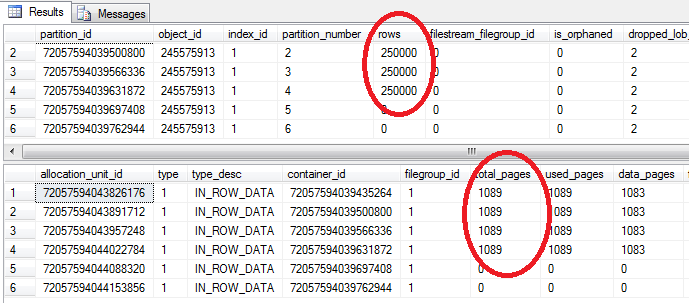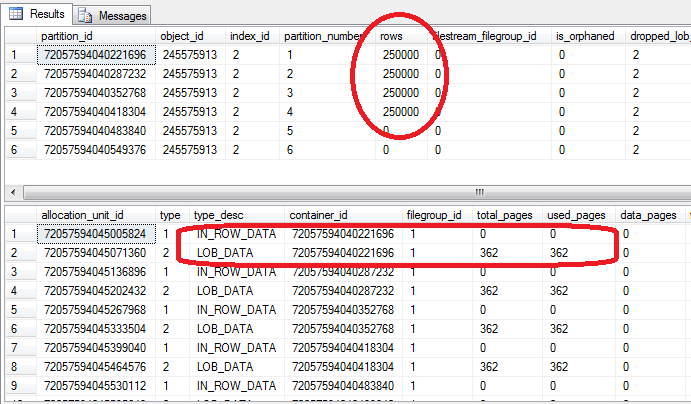# How to use columnstore indexes in SQL Server

July 13th, 2011

Column oriented storage is the data storage of choice for data warehouse and business analysis applications. Column oriented storage allows for a high data compression rate and as such it can increase processing speed primarily by reducing the IO needs. Now SQL Server allows for creating column oriented indexes (called COLUMNSTORE indexes) and thus brings the benefits of this highly efficient BI oriented indexes in the same engine that runs the OLTP workload. The syntax for creating columnstore indexes is described on MSDN at CREATE COLUMNSTORE INDEX. Lets walk trough a very simple example of how to create and use a columnstore index. First lets have a dummy sales table:

``````
create partition function pf (date) as range left for values
('20110712', '20110713', '20110714', '20110715', '20110716');
go

create partition scheme ps as  partition pf all to ([PRIMARY]);
go

create table sales (
[id] int not null identity (1,1),
[date] date not null,
itemid smallint not null,
price money not null,
quantity numeric(18,4) not null)
on ps([date]);
go

create unique clustered index cdx_sales_date_id on sales ([date], [id]) on ps([date]);
go
``````

Notice how I created this table on a partitioning scheme that has one partition a day. See my follow up article How to update a table with a columnstore index to understand why I choose this particular arrangement. For now, lets populate the table with 1 million ‘sales’ facts:

``````
set nocount on;
go

declare @i int = 0;
begin transaction;
while @i < 1000000
begin
declare @date date = dateadd(day, @i /250000.00, '20110712');
insert into sales ([date], itemid, price, quantity)
values (@date, rand()*10000, rand()*100 + 100, rand()* 10.000+1);
set @i += 1;
if @i % 10000 = 0
begin
raiserror (N'Inserted %d', 0, 1, @i);
commit;
begin tran;
end
end
commit;
go
``````

If we look now at the structure of the sales table we see that each partition has 250k rows spread along 1089 pages:

``````
select * from sys.system_internals_partitions p
where p.object_id = object_id('sales');

select au.* from sys.system_internals_allocation_units au
join sys.system_internals_partitions p
on p.partition_id = au.container_id
where p.object_id = object_id('sales');
go
``````If we now run a BI type of query like get the number of sales facts and the total sales for a day, the query would have to scan an entire partition, generating 1089 logical reads:

``````
set statistics io on;
select count(*), sum(price*quantity) from sales where date = '20110713'
set statistics io off;
go

``````

So lets create a columnstore index on this table:

``````
create columnstore index cs_sales_price on sales ([date], price, quantity) on ps([date]);
go
``````

If we look at the structure of the columnstore index we'll see that it has a much smaller footprint, only 362 pages:

``````
select * from sys.system_internals_partitions p
where p.object_id = object_id('sales')
and index_id = 2;

select au.* from sys.system_internals_allocation_units au
join sys.system_internals_partitions p
on p.partition_id = au.container_id
where p.object_id = object_id('sales')
and index_id = 2;
go
``````Note how the columnstore index has no pages allocated for the IN_ROW_DATA allocation unit, but instead has pages allocated to the LOB_DATA allocation unit. So a columnstore index has no rows, instead it uses the BLOB storage to store the column 'segments'. Due to compression possible with column oriented storage, it needs only about one third of the pages needed by the clustered index, although it contains the same columns and the same number of sales facts. If we run again the very same query as before, we'll see how it uses the columnstore index and generates less IO:

``````
set statistics io on;
select count(*), sum(price*quantity) from sales where date = '20110713'
set statistics io off;
go